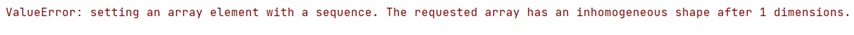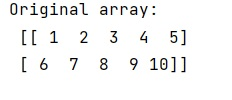# How to fix 'ValueError: The requested array has an inhomogeneous shape after 1 dimensions'?

Learn, how can we fix 'ValueError: The requested array has an inhomogeneous shape after 1 dimensions'?
Submitted by Pranit Sharma, on March 08, 2023

## 'ValueError: The requested array has an inhomogeneous shape after 1 dimensions' – How to fix?

Suppose we are working on N-D arrays and we are creating different rows for an N-D array. Many times, we face this ValueError that says that the requested array has an inhomogeneous shape after 1 dimensions.

This type of error occurs because we do not put an equal number of elements in each row. To solve this problem, we must put an equal number of values in all the rows of an ND numpy array.

Let us understand with the help of an example,

### Example

```# Import numpy
import numpy as np

# Creating a numpy array
arr = np.array([[1,2,3,4,5],[6,7,8,9]])

# Display original array
print("Original array:\n",arr,"\n")
```

### Output## Python code to fix "The requested array has an inhomogeneous shape after 1 dimensions" Error

```# Import numpy
import numpy as np

# Creating a numpy array
arr = np.array([[1,2,3,4,5],[6,7,8,9,10]])

# Display original array
print("Original array:\n",arr,"\n")
```

### OutputLanguages: » C » C++ » C++ STL » Java » Data Structure » C#.Net » Android » Kotlin » SQL
Web Technologies: » PHP » Python » JavaScript » CSS » Ajax » Node.js » Web programming/HTML
Solved programs: » C » C++ » DS » Java » C#
Aptitude que. & ans.: » C » C++ » Java » DBMS
Interview que. & ans.: » C » Embedded C » Java » SEO » HR
CS Subjects: » CS Basics » O.S. » Networks » DBMS » Embedded Systems » Cloud Computing
» Machine learning » CS Organizations » Linux » DOS
More: » Articles » Puzzles » News/Updates